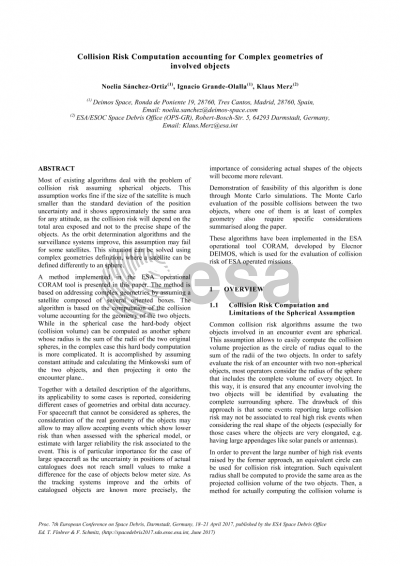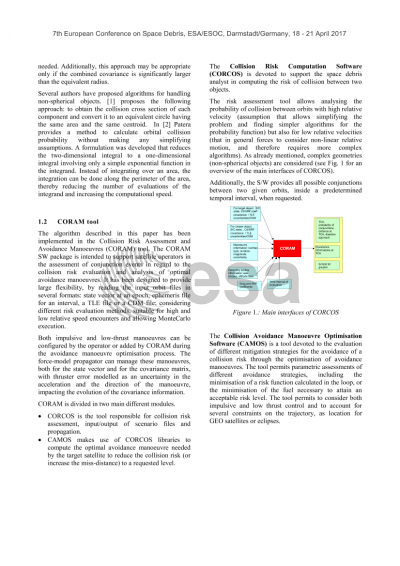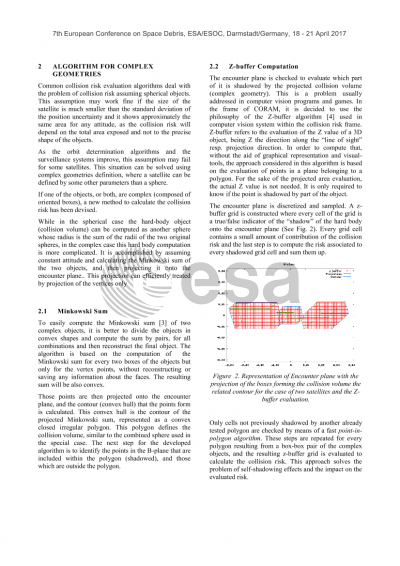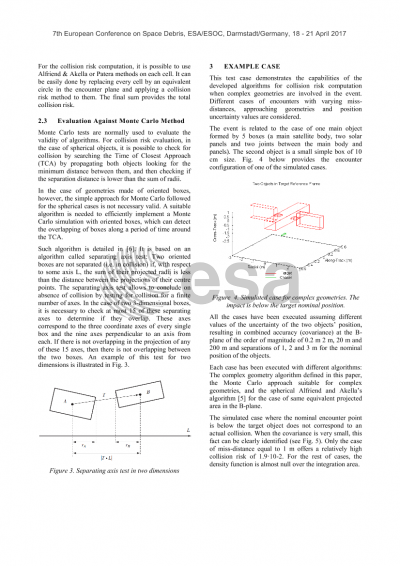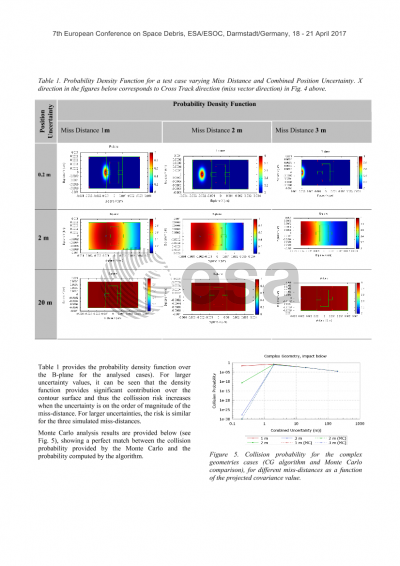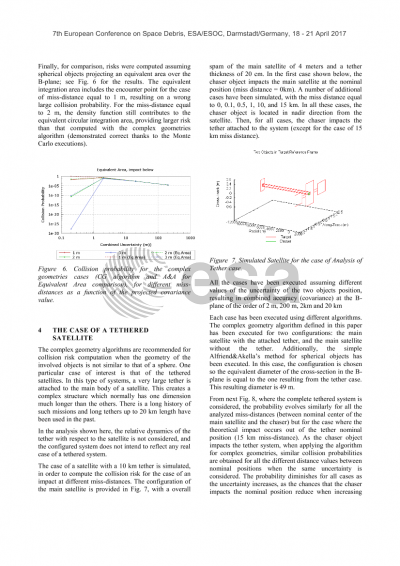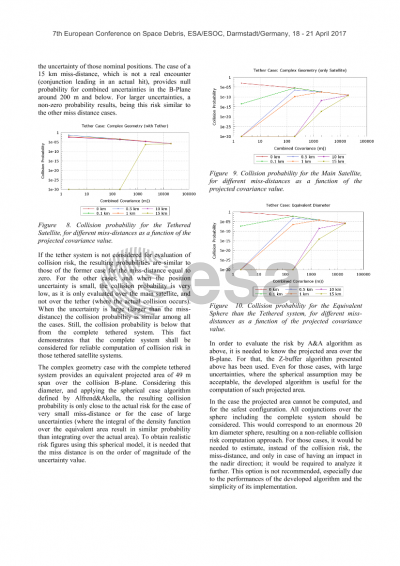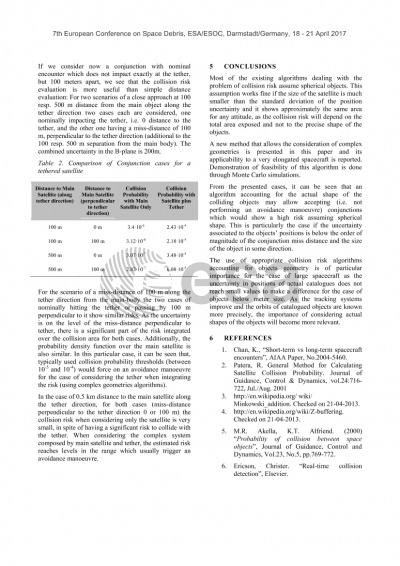# Collision Risk Computation accounting for Complex geometries of involved objects

Noelia Sánchez-ortiz1
Elecnor DEIMOS1

## Document details

Publishing year2017 PublisherESA Space Debris Office Publishing typeConference Name of conference7th European Conference on Space Debris
Pagesn/a Volume
7
Issue
1
Editors
T. Flohrer, F. Schmitz
Download paper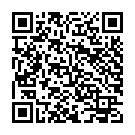## Abstract

Most of existing algorithms deal with the problem of collision risk assuming spherical objects. This assumption works fine if the size of the satellite is much smaller than the standard deviation of the position uncertainty and it shows approximately the same area for any attitude, as the collision risk will depend on the total area exposed and not to the precise shape of the objects. As the orbit determination algorithms and the surveillance systems improve, this assumption may fail for some satellites. This situation can be solved using complex geometries definition, where a satellite can be defined differently to an sphere.
A method implemented in the ESA operational CORAM tool is presented in this paper. The method is based on addressing complex geometries by assuming a satellite made by several oriented boxes. The algorithm is based on the computation of the collision volume accounting for the geometry of the two objects. While in the spherical case the hard-body object (collision volume) can be computed as another sphere whose radius is the sum of the radii of the two original spheres, in the complex case this hard body computation is more complicated. It is accomplished by assuming constant attitude and calculating the Minkowski sum of the two objects, and then projecting it onto the encounter plane. Additionally, the collision volume is translated to the B-plane, by means of the projection of the vertices of such volume.
Together with a detailed description of the algorithms, its applicability to some cases is reported, considering different cases of geometries and orbital data accuracy. For spacecrafts that cannot be considered as spheres, the consideration of the real geometry of the objects may allow to discard events which are not real conjunctions, or estimate with larger reliability the risk associated to the event. This is of particular importance for the case of large spacecrafts as the uncertainty in positions of actual catalogues does not reach small values to make a difference for the case of objects below meter size. As the tracking systems improve and the orbits of catalogued objects are known more precisely, the importance of considering actual shapes of the objects will become more relevant.
Demonstration of feasibility of this algorithm is done through Monte Carlo simulations. The Monte Carlo evaluation of the possible collisions between the two objects, where one of them is at least of complex geometry also require specific considerations summarised along the paper.
Application of this algorithm for the case of high and low relative velocity encounters will be described.
These algorithms have been implemented in the ESA operational tool CORAM, developed by DEIMOS, which is used for the evaluation of collision risk of ESA operated missions.

## Preview